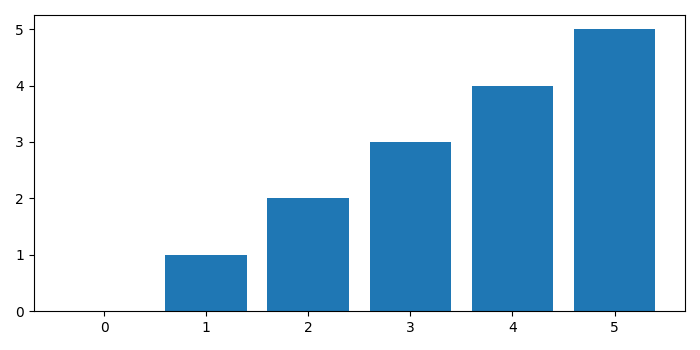# How to plot a bar chart for a list in Python matplotlib?

To plot a bar chart for a list in python matplotlib we can take the following steps.

## Steps

• Set the figure size and adjust the padding between and around the subplots.

• Make a list of data points.

• Make a bar plot with data.

• To display the figure, use Show() method.

## Example

from matplotlib import pyplot as plt

# Set the figure size
plt.rcParams["figure.figsize"] = [7.00, 3.50]
plt.rcParams["figure.autolayout"] = True

# List of data points
data = [0, 1, 3, 2, 1, 5, 2, 1, 4, 2, 4, 0]

# Plot bar chart with data points
plt.bar(data, data)

# Display the plot
plt.show()


## Output

It will produce the following output −